# Research on Performance of Finned Tube Bundles of Indirect Air-cooled Heat Exchangers

Research on Performance of Finned Tube Bundles of Indirect Air-cooled Heat Exchangers

Wei Zhang Xiaoze DuLijun Yang Yongping Yang

Key Laboratory of Condition Monitoring and Control for Power Plant Equipment (North China Electric Power University), Ministry of Education, Beijing 102206, China

Corresponding Author Email:
zhangwei@ncwu.edu.cn
Page:
47-51
|
DOI:
https://doi.org/10.18280/mmep.030108
N/A
|
Accepted:
N/A
|
Published:
31 March 2016
| Citation

OPEN ACCESS

Abstract:

The application of indirect air-cooled condensers in power plants has been increasing rapidly. Indirect air-cooled heat exchangers are the basic equipment. It is of great importance to identify the thermo-flow performance of finned tube bundles of heat exchangers of indirect air cooling systems. The thermo-flow performance of indirect air cooling tube bundles are get by numerical simulation. The correlation of pressure drop and heat transfer coefficient with air velocity are arrived. The dimensionless characteristic number correlations of the friction factor and Nusselt number against the Reynolds number are fitted. We also introduce PEC to evaluate the comprehensive performance of flow and heat transfer performance. The results show that air-side heat transfer coefficient and pressure drop increase with the windward air velocity, in which heat transfer coefficients are more sensitive to change of air velocities than pressure drop. Compared with the experimental results, numerical simulation results match experimental results well. During the same windward air velocity, the simulated heat transfer coefficient is 4.30%~15.76% higher than the experimental results and simulated resistance is higher 2.57% ~14.01% than the experimental results.

Keywords:

indirect air cooling heat exchanger, four-row finned tube bundles, flow and heat transfer performance, numerical simulation

1. Introduction

The application of indirect air-cooled condensers in power plants has been increasing rapidly throughout countries where water resources are in shortage. There are many benefits in indirect air cooling systems such as low operating back pressure, high resistance to the strong wind and low operation noise etc. Since 2010 the SCAL indirect air cooling systems has been widely used in many indirect air cooling projects in which the surface condenser is used and the aluminium or steel heat exchangers are vertical arranged outside the cooling tower circumferentially. For great specific heat capacity variation of the air and water, the finned tube bundles are widely used in heat exchangers of indirect cooling systems. It is of great importance to analyze the thermo-flow performance of the heat exchangers for the optimized design and highly efficient operation of the air cooling system.

About the performance of different types of fined tube bundles, many researches has made. Rocha  et al. acquired the heat exchanging coefficient of the finned tube bundles by experimental analysis of heat exchangers with flat finned round tubes and elliptical tubes. Halici  et al. studied the influence of the row numbers of the flat finned heat exchangers on its flow and heat transfer performance. Saboya  et al. identified the average heat exchanging coefficient of the plate-finned elliptical tube heat exchangers by using naphthalene sublimation. He  et al. analyzed the numerical simulation results of the flat finned tube bundles by using field synergy principle and found the influence of the Reynolds number and parameters of the fins on the heat exchanging performance of tube bundles. Mon and Gross  performed the numerical research and flow field visualization experiments to the performance of the four-row angular fined tube bundles both in staggered arrangement and in-line arrangement. Yan and Sheen  attained the correlations between the convection coefficient and pressure drop versus the windward wind velocity by researching the pressure drop and heat exchanging performance of heat exchangers with flat finned, wave finned and louver finned tube bundles. Ibrahim and Gomaa  et al. investigated the flow and heat transfer performance of the elliptical tube bundles during being brushed vertically and simulated the flow and heat transfer performance in both staggered and in-line arrangement. Liu Fei and He Guogeng  performed the numerical simulation on the air side wind resistance of the multi-row finned tube bundles and analyzed the rules of the resistance versus different inlet wind velocity. Wang et al.  performed experimental research to the air side heat exchanging performance of 18 types of popular tube bundles in the wind tunnel. Kim  et al. studied the heat transfer performance of 22 types of flat finned tube heat exchangers in different fin pitch, rows and arrangement. Dang Yanhui  analyzed the distribution rules of the heat transfer coefficient on the rectangle fin surface when there is or not disturbing holes on fins. The research of Tiwari  et al. showed that vortex generator can increase the performance of the elliptical tube heat exchangers significantly. In conclusion, there is lots of research on flow and heat transfer performance of finned tube heat exchangers. However, there are few researches on the indirect air cooling heat exchangers in the power plants and the experimental models are extremely simplified. The study intends to get the performance of the widely used Forgo type heat exchangers by numerical simulation and experiment and provide reference for design and optimization of ACC.

2. Physical and Mathematics Model

2.1 Physical model of basic indirect cooling heat exchanging elements

There are many indirect air cooling heat exchanger (IDCR) manufacturers. For the different systems there is great difference in the material, tube type and thickness, tube distance, manufacturing method, fin thickness and pitch, fin ration, arrangement and flow numbers of the heat exchangers manufactured by different manufacturers. The products of GEA-EGI are mainly Forgo 4th~6th generation heat exchangers. Among them, the fourth and fifth generation heat exchangers are one-pass flow and usually arranged vertically and circumferentially outside of the tower. The latest Forgo sixth generation heat exchangers are four-row, two-pass flow and arranged vertically and circumferentially outside of the tower. The latest four-row tube heat exchangers are composed of round aluminum tubes & aluminum fins , which are chosen as the typical indirect air cooling heat exchanger model in the experiment whose outline drawing is shown in Fig. 1. It is composed of base tubes and fins with the air flowing outside. The diameter of the heat exchanger base tube is 25 mm with the thickness is 1.5 mm.

## 1.png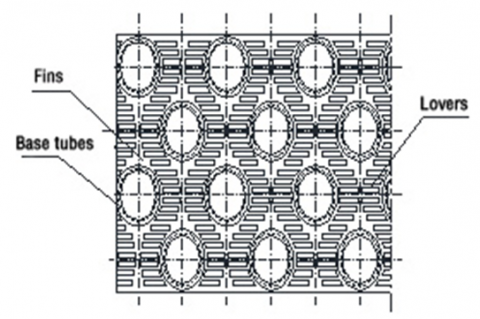Figure 1. Outline drawing of four-row finned tube bundles

2.2 Mathematics model and boundary conditions

Base tubes of the indirect air cooling heat exchangers exchange heat of water inside the tubes to the aluminium fins via heat conduction, then the base tube and fins transfer heat to the outside air by convection. Therefore, the heat transfer of four-row tube bundles is the coupling of convection via air and heat transfer via solid. For the periodicity and symmetry in the geometry of the heat exchangers, a symmetrical part of the tube bundles is selected and the model is built as shown in Fig. 2.

## 2.png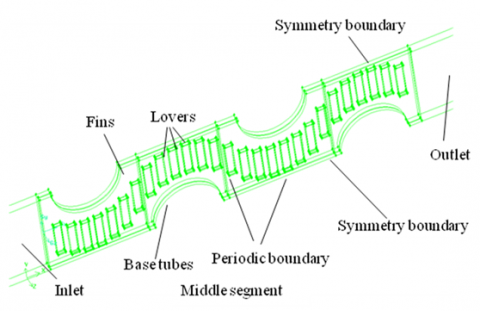Figure 2.  Computational model and boundary conditions

The whole computational domain is divided into the inlet segment, middle segment and outlet segment. To ensure the computational precision and save the computer’s calculating capability, the non-uniform hexahedral structural grid is used. The simulation is performed in different grid quantities to ensure the simulation result is not influenced by the grid quality and check the grid-independent of the result . Finally, the total amount of grids in the model is decided as 10, 607, 540.

The velocity inlet boundary conditions are used in the computational domain. The air windward velocity is 0.5~2.4m/s and the inlet air temperature is 25.5oC. The pressure outlet boundary conditions are used in the outlet. The periodic boundary conditions are used in the up and down direction and the symmetry boundary conditions are used in the forward and backward direction. RNG k-ε is used in this turbulence model. SIMPLE method is used in the coupling of pressure and velocity.

Regarding the flow as steady, incompressible, gravity neglecting, constant physical property, the control equation of flow and heat transferring refers to the documents. Under the conditions of ambient air pressure is 1atm, the flow and heat transferring of the air outside of the indirect air cooling heat exchanger tubes is simulated. Thermal resistance in water side, contact resistance between fins and tubes and the fouling resistance during real operation are neglected.

2.3 Flow field and temperature field

Fig.3 shows the contour of the flow field, temperature field and pressure field of the protruded finned four-row round-tube bundles. It can be shown in the figure that the air velocity increases due to the reduction of flow section when the air flows around the round tubes but becomes nearly uniform in the flow region because of the function of disturbing holes. Furthermore, on both ends of the disturbing holes, the velocity is higher because of the higher edge effect and the trailing vortex in the rear part of the base tubes is improved. Based on the disturbance and mixed-effect of the disturbing holes to the boundary layer, the distribution of temperature on the whole fin is improved. As a result, the average heat transferring performance of the fined tube bundles increases.

## 3.png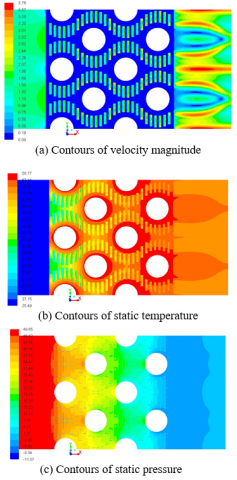Figure 3. Contours of velocity, temperature and pressure

2.4 Experimental result validation

The thermo-flow performance of tube bundles is performed in North China Electric Power University by using wind tunnels. The experiment facilities, accuracy of instruments, data processing and the results can be referred in the documents . And the data is also processed by complying with the experimental data of the heat and resistance in the air cooling heat exchanger laboratory regulated in the testing code of the dry cooling and condenser of the thermal power plant (DL/T 552-95).

3. Results and Discussion

3.1 Data processing

The pressure difference ($\Delta p$) between inlet and outlet is:

$\Delta p=p_{\text {in}}-p_{\text {out}}$.                                                                    (1)

The total surface heat transfer coefficient in the air side is:

$h=\frac{Q}{A \Delta T_{m}}$.                                                                          (2)

where Q is heat transfer rate, A is the outside surface area of the finned tube bundle model and $\Delta T_{m}$ is the logarithmic mean temperature difference as follows:

$\Delta T_{m}=\frac{\left(T_{t}-\bar{T}_{i n}\right)-\left(T_{t}-\bar{T}_{\text {out}}\right)}{\ln \left[\left(T_{t}-\bar{T}_{i n}\right) /\left(T_{t}-\bar{T}_{\text {out}}\right)\right]}$                 (3)

where $T_{t}$ is the wall temperature of the base tube, $\bar{T}_{i n}$, $\bar{T}_{o u t}$ are the inlet and outlet average air temperatures.

The Reynolds number of the air side is:

$R e=\frac{u D}{v}$                                                                              (4)

The Nusselt number (Nu) and friction coefficient (f) is is:

$P E C=N u / f^{1 / 3}$                                                                   (5)

$P E C=N u / f^{1 / 3}$                                                                   (6)

where u is the velocity on the minimum flow section between the fins, D is the characteristic length and the outer diameter of the tubes is used, v is the air kinematic viscosity, $\rho$ is the average air density and $\lambda$ is the air heat transfer coefficient.

Performance evaluation criteria (PEC) is selected as the comprehensive flow and heat transfer performance critia. Higher PEC means better thermo-flow performance.

$P E C=N u / f^{1 / 3}$                                                                   (7)

3.2 Analysis of the numerical simulation results

According to the simulation results, the rules of the pressure drop and heat transfer coefficient versus the changing of air windward velocities is shown in Fig.4. With the increasing of windward air velocity, the flow resistance and heat transfer coefficient of the four-row finned tube bundles increased accordingly.

The increase of heat transfer coefficient is much higher than that of pressure drop. Comparisons between simulation and experimental values they agree very well. At the same windward velocity, the simulated heat transfer coefficient is 4.30%~15.76% higher than the experimental values and the simulated resistance is 2.57%~14.01% higher than the experimental values. The rule of the calculated friction factor and Nusselt number versus the variation of Reynolds number is shown in Fig.5. It indicates that f decreased significantly with the increasing Re especially at low Re. On the contrary, Nu increases with the increasing Re.

## 4.png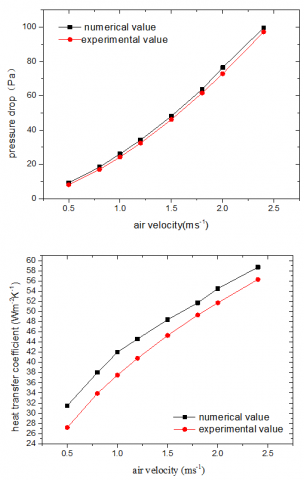Figure 4.  Air pressure drop and heat transfer coefficient at different air velocities

## 5.pngFigure 5.  Friction factor and Nusselt number versus Reynolds number

PEC shows the comprehensive thermo-flow performance directly. It can be found in Fig.6 that PEC increases with Re which means the  comprehensive thermo-flow performance of the finned tubes is strengthened, i.e. the increase of heat transfer performance is higher than the increase of flow resistance.

## 6.png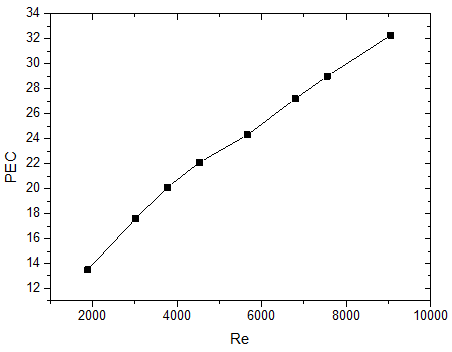Figure 6.  PEC versus Reynolds number

3.3 Characteristic number correlation

The dimensionless coefficient correlation of the friction factor and Nusselt number versus the Reynolds number is shown in equation (8)~(10) which is fitted from the numerical simulation of the correlation of the pressure drop, heat transfer coefficient and PEC versus the variation of the windward wind velocity. They can be applied to the design and check of the indirect air cooling heat exchangers.

$f=335.92 \mathrm{Re}^{-0.475}$.                                                              (8)

$N u=1.529 \mathrm{Re}^{0.3885}$.                                                              (9)

$P E C=0.22 \mathrm{Re}^{0.5467}$.                                                           (10)

The correlation is adaptable in the range of 1000≤Re≤18000.

4. Conclusions

By the numerical simulation of the flow and heat transfer performance of the indirect air cooling tube bundles, the rules of the flow resistance and heat transfer coefficient against the variation of windward velocity of the four row air cooling tube bundles are acquired. Then, the dimensionless correlations of the friction factor and Nusselt number against the Reynolds number are fitted which can be used in the design and check of the indirect air cooling system. By data analyzing and comparison with test, it can be found that the heat transfer performance and pressure drop of the four-row heat exchangers increases together with the increasing windward air velocity and the comprehensive thermo-flow performance enhanced. Numerical simulation results match well experimental results.

References

 L.A.O. Rocha, F.E.M. Saboya, J.V.C. Vargas, “A comparative study of elliptical and circular sections in one and two row tubes and plate fin heat exchangers, ” Int J Heat Fluid Flow, vol. 18, no. 2, pp. 247-252, Apr. 1997. DOI: 10.1016/S0142-727X(96)00063-X.

 Halici F., Taymaz I., Cunduz M., “The effect of the number of tube rows on heat, mass and momentum transfer in flat-plate finned tube heat exchangers, ” Energy, vol. 26, no. 11, pp. 963-972 , Nov. 2001. DOI: 10.1016/S0360-5442(01)00048-2.

 S. M. Saboya, F.E.M. Saboya, “Experiments on elliptic sections in one and two row arrangements of plate fin and tube heat exchangers, ” Exp. Therm. Fluid Sci., vol. 24, no. 1-2, pp. 67-75, Mar. 2001. DOI: 10.1016/S0894-1777(00)00059-5.

 He Y. L., Tao W. Q., Song F. Q., et al., “Three-dimensional numerical study of heat transfer characteristics of plain plate fin-and-tube heat exchangers from view point of field synergy principle, ” Int. J. Heat Fluid Flow, vol. 26, no. 3, pp. 459-473,  June 2005. DOI: 10.1016/j.ijheatfluidflow.2004.11.003.

 Mon M. S., Gross U., “Numerical study of fin-spacing effects in annular-finned tube heat exchangers, ” Int. J. Heat Mass Transf., vol. 47, no. 8-9, pp. 1953-1964, Apr. 200. DOI: 10.1016/j.ijheatmasstransfer.2003.09.034.

 Yan W. M., Sheen P. J., “Heat transfer and friction characteristics of fin-and-tube heat exchangers,” Int. J.  Heat Mass Transf., vol. 43, no. 9, pp. 1651-1659, May 2000. DOI: 10.1016/S0017-9310(99)00229-X.

 Ibrahim T. A., Gomaa A, “Thermal performance criteria of elliptic tube bundle in cross flow, ” Int. J. Therm. Sci., vol. 48, no. 11, pp. 2148-2158, Nov. 2009. DOI: 10.1016/j.ijthermalsci.2009.03.011.

 Liu Fei, He Guogeng, “Numerical simulation of finned-tube air cooler with many rows,” Refrigeration and Air-Conditioning, vol. 4, no. 4, pp. 30-33, 2004.

 Wang C. C., Chi K. Y., “Heat transfer and friction characteristics of plain fin-and-tube heat exchangers, Part I: new experimental data, ” Int. J. Heat Mass Transf., vol. 43, no. 15, pp. 2681-269, Aug. 2000. DOI: 10.1016/S0017-9310(99)00332-4.

 Kim Y., “Heat transfer characteristics of flat plate finned-tube heat exchangers with large fin pitch, ” Int. J. Refrig., vol. 28, no. 6, pp. 851-858, Sept. 2005. DOI: 10.1016/j.ijrefrig.2005.01.013.

 Dang Yanhui, Ming Tingzhen, Liu Wei et al., “Numerical simulation on flow and heat transfer characteristics of rectangle-wing elliptic tube with      interrupted holes, ” CIESC Journal, vol. 60, no. 12, pp. 2975-2980, 2009.

 Tiwari S., Maurya D., Biswas G., et al., “Heat transfer enhancement in cross-flow heat exchangers using oval tubes and multiple delta winglets, ” Int. J. Heat Mass Transf., vol. 46, no. 15, pp. 2841-2856, July 2003. DOI: 10.1016/S0017-9310(03)00047-4.

 Zsolt Forray, “State-of-art Forgo type heat exchangers-the latest development, ” Proceedings of the national conference on air cooling system, China Electricity Council, Yinchuan City, Ningxia Province, 2009. DOI:

 P. J. Roache, “Perspective: a method for uniform reporting of grid refinement studies, ” Trans. ASME, J. Fluids Eng., vol. 116, no. 3, pp. 405-413, Sept. 1994.  DOI: 10.1115/1.2910291.

 North China Electric Power University, Report of the heat transfer and resistance performance test on the four-row heat exchanging elements with aluminum tubes and aluminum fins, 2011.